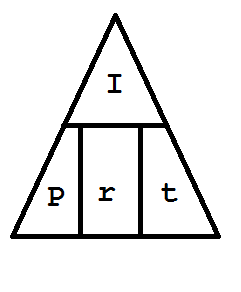# Simple Interest

Introduction

## Have your ever promised a date on payment of borrowed money?

### Definition of terms:

Lender or creditor- a person who invests money or makes finances available for investment.

Borrower or debtor- a person who owes money to a lender or receives funds from one.

Origin or loan date- the date on which the borrower receives the funds.

Repayment date or maturity date- when the money borrowed or loan is due to be paid back.

Time or term (t)- the length of time in years that money is borrowed or invested; the time between the date of origin and the date of maturity.

Principles (P)- on the origin date, the amount borrowed or invested.

Rate (r)- the lender's annual rate, commonly expressed in percent, or the investment's rate increase.

Interest (I)- amount paid or earned for the use of money.

Simple Interest- interest is calculated on the principal and added to it.

Maturity value or Future value (F)- amount that the lender receives from the borrower on maturity data after years.

At the end of the lesson, the learner is able to compute interest, maturity value and present value in simple interest environment, and solve problems involving simple interest

Process

## Annual Simple Interest

I = Prt

where :                   I = simple interest

P = principal or amount invested or borrowed

r = simple interest rate

t = terms or time in years

Example 1: A bank offers 0.25% annual simple interest rate for a particular deposit. How much interest will be earn if one million pesos is deposited in this savings account for 1 year?
Given : P = ₱ 1,000,000.00             r = 0.25% = 0.0025                 t = 1

Find : Interest

Solution : I = Prt

I = (₱ 1,000,000.00) (0.0025) (1)

I = (₱ 1,000,000.00) (0.0025)

I = ₱ 2,500.00

Answer : The interest earned is ₱ 2,500.00

Example 2: How much interest is charged when ₱ 50,000.00 is borrowed for 9 months at an annual rate of 10%?

Given : P = ₱ 50,000.00                r = 10% = 0.10                    t = 9/12 = 0.75   Note: when the terms is expressed in months (M),

Find : Interest                                                                                                                 it should be converted in years by t = M/12

Solution : I = Prt

I = (₱ 50,000.00) (0.10) (0.75)

I = (₱ 5, 000.00) (0.75)

I = ₱ 3,750.00

Answer : The simple interest charged is ₱ 3,750.00

Example 3 : Complete the table by finding the unknown.

 Principal     (P) Rate   (r) Time   (t) Interest     (I) (a) 2.5% 4 ₱ 1,500.00 ₱ 36,000.00 (b) 1.5 ₱ 4,860.00 ₱ 250,000.00 0.5% (c) ₱ 275.00 ₱ 500,000.00 12.5% 10 (d)

Solution:(a) The unknown principal can be obtained by :

Given : r = 2.5% = 0.025                 t = 4                 I = ₱ 1,500.00

Find: Principal

Solution :       P = I/rt

P = ₱1,500.00/ (0.025) (4)

P = ₱1,500.00/ 0.1

P = ₱ 15,000.00

Answer : The principal is ₱15,000.00

(b) The unknown rate can be obtain by :

Given : P = ₱ 36,000.00                 t = 1.5            I = ₱ 4,860.00

Find : rate

Solution :     r = [I/Pt] x 100%

r = [₱ 4,860.00/ (₱ 36,000.00) (1.5)] x 100%

r =  [₱ 4,860.00/ ₱54,000.00] x 100%

r = 0.09 x 100%

r = 9%

Answer : The rate is 9%

(c) The unknown time can be obtain by :

Given : P = ₱ 250,000.00            r = 0.5% = 0.005          I = ₱ 275.00

Find : time

Solution :     t = I/Pr

t = ₱ 275.00/ (₱ 250,000.00) (0.005)

t =  ₱ 275.00/ ₱ 1,250

t = 0.22 years

Answer : The time is 0.22 years

(d) The unknown interest can be obtain by:

Given : P = ₱ 500,000.00            r = 12.5% = 0.125          t = 10

Find: Interest

Solution :     I = Prt

I = ( ₱ 500,000.00) (0.125) (10)

I = ( ₱ 500,000.00) (1.25)

I = ₱ 625,000.00

Answer : The interest is ₱ 625,000.00

For clarification discussion please watch the video

Evaluation

A. Find the unknown principal (P) , rate (r), time (t) and interest (I) by completing the table. Show your complete solution and send it on my messenger

 Principal (P) Rate (r) Time (t) Interest (I) ₱ 2,000.00 5% 3 (1) (2) 12% 5 ₱ 20,000.00 ₱ 88,000.00 (3) 4 ₱ 8,000.00 ₱ 500,000.00 9.5% (4) ₱ 285,000.00 ₱ 1,000,000.00 0.5% 12 (5)

B. Solve the following problems. Show your complete solution and send it on my messenger

6. Find the simple interest on a loan of ₱ 65,000.00 if the loan is given at a rate of 20% and is due in 3 years.

7. Jake invested a certain amount at 10% simple interest per year. After 2 years, the interest she received amounted to ₱ 3,000.00. How much did she invest?

8. Miko borrowed ₱ 25,000.00 at 10% annual simple interest rate. How much should he pay after 3 years and 6 months?

9. How long will an amount of ₱ 50,00.00 gain a simple interest of ₱ 10,000.00 at 4% per annum?

10. At what simple interest rate per annum will ₱ 20,000.00 accumulate to ₱ 25,000.00 in 3 years?

Credits

Reference: General Mathematics Learners Material by Department of Education Republic if the Philippines

Teacher Page

Im Sir Jake Magboo